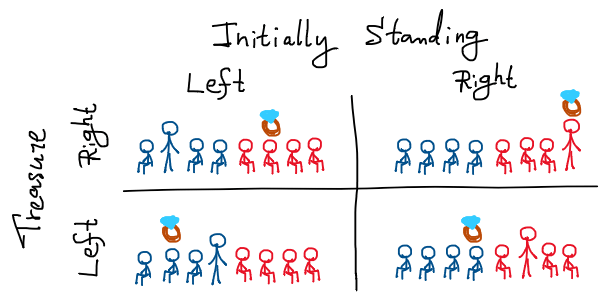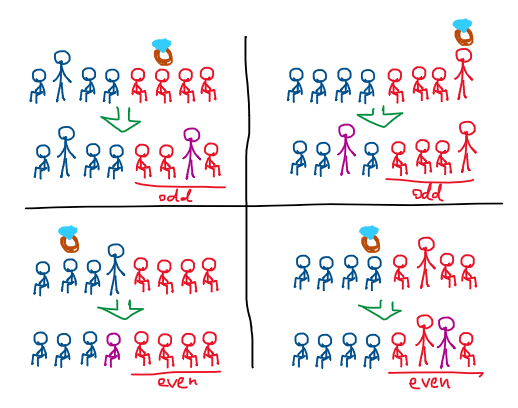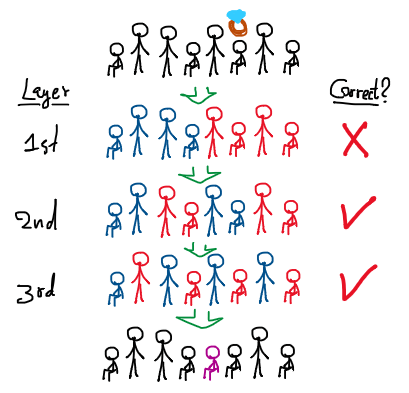# Hint the Hidden Heirloom

Saturday, August 19, 2023, 06:48 AM

Consider a magic trick where 8 volunteers are randomly selected from the audience. The selected volunteers are lined up on the stage, each possessing a stool. The magician picks an object as a treasure, a heirloom maybe (whether it’s a coin, a card, one ring, etc.), and gives it to the group, then leaves the room. The group decides for one person to “hide” the treasure in their pocket, then return to their stool. Now each of them has their own free will to either sit down or just stand still. The magician’s assistant, witnessing the entire event, selects exactly one volunteer and instructs him/her to sit down if he/she is already standing, or vice versa. Afterward, the magician returns to the stage and accurately points out the volunteer who possesses the treasure. (Of course, there’s no mean of communication between the magician and the assistant.)

 treasure? stand? name: A B C D E F G H

### magician

Interactive simulation of this magic trick

How could this magic work? In other words, what is the protocol the magician and the assistant agreed in prior so they can “hint” the right person using only one alteration over a seemingly random information?

Side note: I’ve seen this magic trick countless times. IIRC, the very first time was w/ my com-sci friends. Besides, TED-Ed already covered this riddle with an excellent solution two years ago. However, I find their solution to be too magical. Therefore, I’d like to present you yet another lengthily train-of-thought on how to tackle the problem.

## Trivial case

We may first take a look at the most trivial case. What if everyone sat down initially? The assistant would only need to pick the one with the treasure to stand up. That’s the most straightforward technique. Right?

What if everyone is standing instead? Well, this technique still works, since the assistant can just call the one with the treasure to sit down.

How about only one standing? We can see that this technique is not possible anymore, since the assistant must change one person. So after that either two person standing or no one standing. Either way, the information is loss.

Since we cannot generalize this technique to every initial sit-stand combination, we can’t be sure if the correct solution produce the same result. Still, it’s a good start to have them and we’ll note those two solutions for future use.

## Left or right?

When faced with such challenges, one approach is to take a step back and relax. Both in relaxing our mind and the constraints of the puzzle. What if we don’t need to pin point the one who keep the treasure, for now. But we just only need to tell which side of the stage the treasure belongs to?

Let’s consider the previous unsolved case of one standing volunteer. It is easy to see that there are 4 possibilities initially: the standing person is on either on the left or right side of the stage, and also the treasure.All possibilities when considered only left-right

We only need to categorize the possibilities into 2 groups, after we order one person to stand up/sit down, so now there are 0 or 2 persons standing.

How about we make a rule that the side with persons standing should contains the treasure? We can see that this rule is quite redundant since if there are persons standing on the left side, then there should be no persons stand on the right side, and vice versa. And this rule will be useless when the initial standing person is on the different side of the treasure. Because

1. if we order the standing person to sit down, we lost information (no one standing),
2. if we order another person on the same side to stand up, we give wrong hint, and
3. if we order another person on the different side to stand up, we lost information (both side standing).

So, what if we just check only one side, say, the right side. If we see persons standing on the right side, then we say that the treasure is on the right side, otherwise the treasure is on the left? This rule is just work, since

1. treasure left, standing left: we order another person on the left to stand up,
2. treasure left, standing right: we order the standing person to sit down,
3. treasure right, standing left: we order another one on the right to stand up, and
4. treasure right, standing right: we order another one on the right to stand up.

Let’s check with our trivial case. If everyone sat down, we can just order one on the correct side to stand up. And… uh oh, this rule broke again when everyone is already standing in the first place.

What is the natural way to “fix” this issue? Well, recalled the solution to the case of everyone standing, we let one with treasure sit down. So the side with treasure has odd number of people standing instead.Example alteration that yields a consistent hinting system

Thus, we modify the rule to count how many people standing up on the right side instead. If the number of people is odd, then we say that the treasure is on the right side.

A quick check on this new rule confirms that it solves the case of one person standing initially, as well as those two trivial cases. In fact, it pass every case where arbitrary number of people standing initially. Because no matter the initial configuration, if the right side has an incorrect number of people standing, then we order one person on the right side to stand/sit (and if the right side is correct in the first place, we just order a person on left side instead).

## Recursionization

Now we know how to solve the problem in the relaxed setting, we of course want to generalize it back to the original setting. So our problem is, after we know the correct left/right side, how can we narrow the search space down further more?

We can directly adapt the previous technique right away. That is we would like to split the group of 8 people into two half, then ask which half the treasure belongs to. The main different is that we don’t want to split them into the same previous left/right group.

What is the most natural way, or even the easiest way to do that? If we think recursively, it is obvious that we can split each previous left/right group into another left/right group again. The 2nd layer of left/right group to narrow down the treasure to 2 person.

Of course, we need the 3rd layer to pin-point the person with the treasure.Put it all together for an algorithm

And that’s it. This is the protocol to hint at which person is holding the treasure. To recap the algorithm, let’s us first label the volunteers from left to right with binary number from 000 to 111. Ultimately, we want to find volunteers with label in form of $\underline{abc}$ (here, variables with underline indicate that it is a number with each variable treated as a digit, not a multiplied by juxtaposition). So that we can make alteration and perform revelation.

Our first goal is to find the 3-digit binary number $\underline{\ell mr}$ for the volunteer to sit/stand. The assistant need to do these steps:

1. Count how many standing volunteers whose leftmost digit is 1 (100, 101, 110, 111).
2. For odd count: check if the treasure belongs to this group? If yes then set $\ell$ to 0, otherwise set to 1.
3. For even count: check if the treasure belongs to this group? If yes then set $\ell$ to 1, otherwise set to 0.
4. Repeat step 1-3, but this time consider volunteers whose middle digit is 1, and store the result in variable $m$.
5. Repeat step 1-3, but this time consider volunteers whose rightmost digit is 1, and store the result in variable $r$.
6. Order the volunteer with label $\underline{\ell mr}$ to stand up/sit down.

Our second goal is to find the label $\underline{LMR}$ for the correct person with the treasure. The magician follows this protocol:

1. Count how many standing volunteers whose leftmost digit is 1 (100, 101, 110, 111).
2. If the count is odd, then set $L$ to 1, otherwise set to 0.
3. Repeat step 1-2, but this time consider volunteers whose middle digit is 1, and store the result in variable $M$.
4. Repeat step 1-2, but this time consider volunteers whose rightmost digit is 1, and store the result in variable $R$.
5. Point that the volunteer with label $\underline{LMR}$ must possess the treasure.

## Bitwise XOR scheme

The previous protocol already answer our curiosity on how one can make the magic works. However, it is quite hard to pull off in the real magic since the protocol is a bit complicated. Luckily, there is an equivalent way to do this using exclusive or (XOR, binary operation: $\oplus$) on binary numbers.

We first consider the magician’s protocol (since it is easier). By reusing the 3-digit binary number $\underline{LMR}$ for the correct person who held the treasure, we have these equations for each digit

$\begin{matrix} L &=& \bigoplus\lbrace &&&& {\color{green}X_{100}}, & {\color{blue}X_{101}}, & {\color{indigo}X_{110}}, & \color{purple}X_{111} & \rbrace, \\ M &=& \bigoplus\lbrace && {\color{orange}X_{010}}, & {\color{olive}X_{011}}, &&& {\color{indigo}X_{110}}, & \color{purple}X_{111} & \rbrace, \\ R &=& \bigoplus\lbrace & {\color{red}X_{001}}, && {\color{olive}X_{011}}, && {\color{blue}X_{101}}, && \color{purple}X_{111} & \rbrace, \end{matrix}$

where $X_k$ of value 1 represents that the volunteer $k$ is standing after the assistant order someone to sit/stand. Thus the magician can just compute

\begin{align} \underline{LMR} &= 100{\cdot}L \;\oplus\; 010{\cdot}M \;\oplus\; 001{\cdot}R \\ &= \bigoplus\begin{bmatrix} &&& 100\color{green}X_{100} & 100\color{blue}X_{101} & 100\color{indigo}X_{110} & 100\color{purple}X_{111} \\ & 010\color{orange}X_{010} & 010\color{olive}X_{011} &&& 010\color{indigo}X_{110} & 010\color{purple}X_{111} \\ 001\color{red}X_{001} && 001\color{olive}X_{011} && 001\color{blue}X_{101} && 001\color{purple}X_{111} \\ \end{bmatrix} \\ &= \bigoplus \lbrace {\color{red}001X_{001}}, {\color{orange}010X_{010}}, {\color{olive}011X_{011}}, {\color{green}100X_{100}}, {\color{blue}101X_{101}}, {\color{indigo}110X_{110}}, {\color{purple}111X_{111}} \rbrace \\ &= \bigoplus_k k {\cdot} X_k. \end{align}

In other words, the magician just XOR label of everyone who’s standing up to find to correct person.

We can also simplify assistant’s protocol for $\underline{\ell mr}$ in a similar manner too. Let $x_k$ represent volunteer $k$ is sat/standing before the assistant made an order. Thus, the system of equations are

$\begin{matrix} \ell &=& \bigoplus\lbrace & L, &&&& {\color{green}x_{100}}, & {\color{blue}x_{101}}, & {\color{indigo}x_{110}}, & \color{purple}x_{111} & \rbrace, \\ m &=& \bigoplus\lbrace & M, && {\color{orange}x_{010}}, & {\color{olive}x_{011}}, &&& {\color{indigo}x_{110}}, & \color{purple}x_{111} & \rbrace, \\ r &=& \bigoplus\lbrace & R, & {\color{red}x_{001}}, && {\color{olive}x_{011}}, && {\color{blue}x_{101}}, && \color{purple}x_{111} & \rbrace. \end{matrix}$

Which summarized to

$\underline{\ell mr} = \left( \bigoplus_k k{\cdot}x_k \right) \oplus \underline{LMR}.$

That is the assistant will do everything as same as the magician: XOR everyone who’s standing up. Then make another extra step: XOR the result with the correct person who hid the treasure.

## Multiple way to hint

As a rule of thumb, magic tricks should be performed only once. Otherwise, they are prone to be solved by the audience, making the magic feel less magical… (but it depends; for us mathematicians, knowing the trick makes it feel more magical, right?)

Luckily, this magic trick can be performed more than once. The easiest way is to swap the representation for sit/stand. In the previous protocol, we let stand = 1 and sit = 0. To deceive some audience who think they’ve already figured out the trick, we can flip the protocol to stand = 0 and sit = 1 instead.

And that’s just one way to obscure the trick. In fact, we can choose any permutation for labeling volunteers. Thus, there are $8!$ distinct ways to obfuscate the protocol.

Lastly, the trick can be adapted to work with a different number of volunteers too, as long as the number of volunteers is in form of $2^n$. Why? Well, maybe I’ll leave it as an exercise…

Have fun performing this magic trick IRL 😊

author